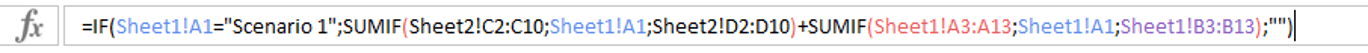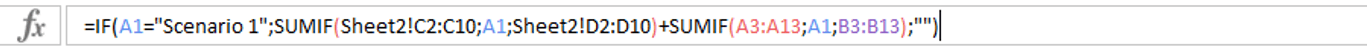# Clean formulas

Modified on Thu, 22 Sep 2022 at 05:10 PM

Excel formulas can frequently become complex, or even very, very complex. When you need to audit your formulas, or if you have to share your Excel spreasheets with other users, it's important to keep formulas as simple as possible.

In many cases, Excel adds useless, redundant information in our formulas. When the user is typing a formula in Sheet1 for instance, Excel may refer to Sheet1!A1 instead or just A1 (which is enough, given that we are already in Sheet1)This often makes your formulas longer and more complex than they actually need to be.

From the Power-user Excel ribbon, click the Clean formulas feature. Power-use rwill run on your current sheet and remove redundant references.

Let's look at the below example.

Here is a formula before applying Clean formulas:And here is the same formula after:As you can see, all references to Sheet1 have been removed because it's a useless redundancy given that the current cell is in Sheet1. However, references to Sheet2 have been kept because they are needed.

This makes the formula shorter and easier to analyze.